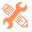## CMS DevelopmentContributor

## Filter HubDB by row using Hubl

SOLVE

I am trying to create a listing page that provides a links by state.  The example below, would show all press releases from California tagged 'CA' in the row 'State'

```{% set table = hubdb_table_rows(679969, queryparam) %}

{% if table == [] %}
<p class='align-center'>Sorry, no listings found for that Search. Try changing your fiter and search again.</p>
{% else %}

{% if row["state"] in table == "CA" %}

<div class="container row-fluid">
<div class="state-post-listing">
<ul>
<li><a href="{{ row['embed'] }}">{{ row["date"]|datetimeformat('%A %e %B %Y') }}&nbsp;|&nbsp;{{ row['name'] }}&nbsp;|&nbsp;{{ row['state'] }}<a/></li>
</ul>
</div>
</div>

{% endif %}

{% endif %}

```
1 Accepted solutionSolution
Contributor

## Filter HubDB by row using Hubl

SOLVE

I did not have the correct logic.  I fixed the problem by adding 'state=CA' where state is the name/ID of the row and CA is the value i am sorting for.

```{% set table = hubdb_table_rows(679969, 'state=CA') %}

{% if table == [] %}
<p class='align-center'>Sorry, no listings found for that Search. Try changing your fiter and search again.</p>
{% else %}

{% for row in table %}

<div class="container row-fluid">
<div class="state-post-listing">
<ul>
<li><a href="{{ row['embed'] }}">{{ row['date'] }} | {{ row['name'] }} | {{ row['state'] }}<a/></li>
</ul>
</div>
</div>

{% endfor %}
{% endif %}```
2 Replies 2HubSpot Product Team

## Filter HubDB by row using Hubl

SOLVESolution
Contributor

## Filter HubDB by row using Hubl

SOLVE

I did not have the correct logic.  I fixed the problem by adding 'state=CA' where state is the name/ID of the row and CA is the value i am sorting for.

```{% set table = hubdb_table_rows(679969, 'state=CA') %}

{% if table == [] %}
<p class='align-center'>Sorry, no listings found for that Search. Try changing your fiter and search again.</p>
{% else %}

{% for row in table %}

<div class="container row-fluid">
<div class="state-post-listing">
<ul>
<li><a href="{{ row['embed'] }}">{{ row['date'] }} | {{ row['name'] }} | {{ row['state'] }}<a/></li>
</ul>
</div>
</div>

{% endfor %}
{% endif %}```